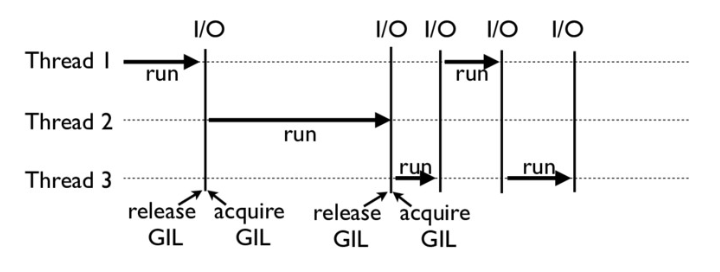1.引言

2.GIL存在的背景

3.GIL主要工作原理

4.Python 线程安全问题

5.可以如何绕过 GIL？

6.延伸阅读

# 1.引言

``import time  def CountDown(n):    while n > 0:        n -= 1  start_time = time.time() n = 100000000CountDown(n) end_time = time.time()print("time cost: {} seconds".format(end_time - start_time))``

``time cost: 5.597759962081909 seconds``

``import timefrom threading import Thread n = 100000000  def CountDown(n):    while n > 0:        n -= 1  start_time = time.time() t1 = Thread(target=CountDown, args=[n // 2])t2 = Thread(target=CountDown, args=[n // 2])t3 = Thread(target=CountDown, args=[n // 2])t4 = Thread(target=CountDown, args=[n // 2])t1.start()t2.start()t3.start()t4.start()t1.join()t2.join()t3.join()t4.join() end_time = time.time()print("time cost: {} seconds".format(end_time - start_time))``

``time cost: 11.078598022460938 seconds``

# 2.GIL存在的背景

GIL，是最流行的 Python 解释器 CPython 中的一个技术术语。它的意思是全局解释器锁，本质上是类似操作系统的 Mutex（互斥锁）。每一个 Python 线程，在 CPython 解释器中执行时，都会先锁住自己的线程，阻止别的线程执行。

``>>> import sys>>>>>> a = []>>> b = a>>> sys.getrefcount(a)3``

• 一是设计者为了规避类似于内存管理这样的复杂的竞争风险问题（race condition）；
• 二是因为 CPython 大量使用 C 语言库，但大部分 C 语言库都不是原生线程安全的（线程安全会降低性能和增加复杂度）。

# 3.GIL主要工作原理# 4.Python 线程安全问题

``import threading n = 0  def foo():    global n    n += 1  threads = []for i in range(100):    t = threading.Thread(target=foo)    threads.append(t) for t in threads:    t.start() for t in threads:    t.join() print(n)``

``>>> import dis>>> dis.dis(foo)LOAD_GLOBAL              0 (n)LOAD_CONST               1 (1)INPLACE_ADDSTORE_GLOBAL             0 (n)``

``n = 0lock = threading.Lock() def foo():    global n    with lock:        n += 1``

# 5.可以如何绕过 GIL？

Python因为存在GIL的问题，感觉不太行 ？其实不需要太沮丧。

Python 的 GIL，是通过 CPython 的解释器加的限制。如果你的代码并不需要 CPython 解释器来执行，就不再受 GIL 的限制。事实上，很多高性能应用场景都已经有大量的 C 实现的 Python 库，例如 NumPy 的矩阵运算，就都是通过 C 来实现的，并不受 GIL 影响。

• 绕过 CPython，使用 JPython（Java 实现的 Python 解释器）等别的实现；
• 把关键性能代码，放到别的语言（一般是 C++）中实现。

# 6.延伸阅读

• Python有可能删除GIL吗？
• Python 字节码bytecode